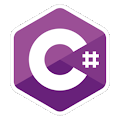Programming Course in C# ¡Free!

# Double, approximation of Pi

Proposed exercise

Create a program to calculate an approximation for PI using the expression

pi/4 = 1/1 - 1/3 + 1/5 -1/7 + 1/9 - 1/11 + 1/13 ...

The user will indicate how many terms must be used, and the program will display all the results until that amount of terms.

Output

Solution

```using System;
public class ApproximationOfPI
{
public static void Main()
{
int n;
double total = 0;

Console.Write( "Enter the amount: " );

for (int i=1; i <= n; i++)
{
int divisor = 2 * i - 1;

if ( i % 2 == 1 )
{
total += 1.0f / divisor;
}
else
{
total -= 1.0f / divisor;
}

Console.WriteLine("Term {0}: {1}", i, 4 * total);
}
}
}
```